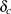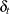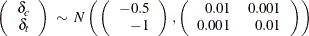# The MI Procedure

### Example 63.18 Adjusting Imputed Values with Parameters in a Data Set

This example illustrates the pattern-mixture model approach in multiple imputation under the MNAR assumption by adjusting imputed values, using parameters that are stored in a data set.

Suppose that a pharmaceutical company is conducting a clinical trial to test the efficacy of a new drug. The trial consists of two groups of equally allocated patients: a treatment group that receives the new drug and a placebo control group. The variable Trt is an indicator variable, with a value of 1 for patients in the treatment group and a value of 0 for patients in the control group. The variable Y0 is the baseline efficacy score, and the variable Y1 is the efficacy score at a follow-up visit.

If the data set does not contain any missing values, then a regression model such ascan be used to test the efficacy of the treatment effect.

Now suppose that the variables Trt and Y0 are fully observed and the variable Y1 contains missing values in both the treatment and control groups. Table 63.11 shows the variables in the data set.

Table 63.11: Variables

Variables

Trt

Y0

Y1

0

X

X

1

X

X

0

X

.

1

X

.

Suppose the data set Mono3 contains the data from the trial that have missing values in Y1. Output 63.18.1 lists the first 10 observations.

Output 63.18.1: Clinical Trial Data

 First 10 Obs in the Trial Data

Obs Trt y0 y1
1 0 10.5212 11.3604
2 0 8.5871 8.5178
3 0 9.3274 .
4 0 9.7519 .
5 0 9.3495 9.4369
6 1 11.5192 13.1344
7 1 10.7841 .
8 1 9.7717 10.8407
9 1 10.1455 10.7279
10 1 8.2463 9.5844

Multiple imputation often assumes that missing values are MAR. Here, however, it is plausible that the distributions of missing Y1 responses in the treatment and control groups have lower expected values than the corresponding distributions of the observed Y1 responses. Carpenter and Kenward (2013, pp. 129–130) describe an implementation of the pattern-mixture model approach that uses different shift parameters for the treatment and control groups, where the two parameters are correlated.

Assume that the expected shifts of the missing follow-up responses in the control and treatment groups,and, have a multivariate normal distributionThe following statements generate shift parameters for the control and treatment groups for six imputations:

proc iml;

nimpute= 6;
call randseed( 15323);
mean= { -0.5 -1};
cov= { 0.01 0.001 , 0.001 0.01};

/*---- Simulate nimpute bivariate normal variates ----*/
d= randnormal( nimpute, mean, cov);

impu= j(nimpute, 1, 0);
do j=1 to nimpute;  impu[j,]= j;  end;
delta= impu || d;

/*--- Output shift parameters for groups ----*/
create parm1 from delta[colname={_Imputation_ Shift_C Shift_T}];
append from delta;
quit;


Output 63.18.2 lists the generated shift parameters in Parm1.

Output 63.18.2: Shift Parameters for Imputations

 Shift Parameters for Imputations

Obs _IMPUTATION_ SHIFT_C SHIFT_T
1 1 -0.56986 -0.90494
2 2 -0.38681 -0.84523
3 3 -0.58342 -0.92793
4 4 -0.48210 -0.99031
5 5 -0.57188 -1.02095
6 6 -0.57604 -1.00853

The following statements impute missing values for Y1 under the MNAR assumption. The shift parameters for the 10 imputations that are stored in the Parm1 data set are used to adjust the imputed values.

proc mi data=Mono3 seed=1423741 nimpute=6 out=outex18;
class Trt;
monotone reg;
mnar adjust( y1 / adjustobs=(Trt='0') parms(shift=shift_c)=parm1)
adjust( y1 / adjustobs=(Trt='1') parms(shift=shift_t)=parm1);
var Trt y0 y1;
run;


The ADJUST option specifies parameters for adjusting the imputed values of Y1 for specified subsets of observations. The first ADJUST option specifies that the shift parameters that are stored in the variable SHIFT_C are to be applied to the imputed Y1 values of observations where TRT=0 for the corresponding imputations. The second ADJUST option specifies that the shift parameters that are stored in the variable SHIFT_T are to be applied to the imputed Y1 values of observations where TRT=1 for the corresponding imputations.

The "Model Information" table  in Output 63.18.3 describes the method that is used in the multiple imputation process.

Output 63.18.3: Model Information

The MI Procedure

Model Information
Data Set WORK.MONO3
Method Monotone
Number of Imputations 6
Seed for random number generator 1423741

The "Monotone Model Specification"  table in Output 63.18.4 describes methods and imputed variables in the imputation model. The MI procedure uses the regression method to impute the variable Y1.

Output 63.18.4: Monotone Model Specification

Monotone Model Specification
Method Imputed Variables
Regression y0 y1

The "Missing Data Patterns"  table in Output 63.18.5 lists distinct missing data patterns and their corresponding frequencies and percentages. The table confirms a monotone missing pattern for these variables.

Output 63.18.5: Missing Data Patterns

Missing Data Patterns
Group Trt y0 y1 Freq Percent Group Means
y0 y1
1 X X X 75 75.00 9.996993 10.655039
2 X X . 25 25.00 10.181488 .

The "MNAR Adjustments to Imputed Values"  table in Output 63.18.6 lists the adjustment parameters for the 10 imputations.

Output 63.18.6: MNAR Adjustments to Imputed Values

MNAR Adjustments to Imputed
Values
Imputed
Variable
Imputation Observations Shift
y1 1 Trt = 0 -0.5699
1 Trt = 1 -0.9049
2 Trt = 0 -0.3868
2 Trt = 1 -0.8452
3 Trt = 0 -0.5834
3 Trt = 1 -0.9279
4 Trt = 0 -0.4821
4 Trt = 1 -0.9903
5 Trt = 0 -0.5719
5 Trt = 1 -1.0209
6 Trt = 0 -0.5760
6 Trt = 1 -1.0085

The following statements list the first 10 observations of the data set Outex18 in Output 63.18.7:

proc print data=outex18(obs=10);
var _Imputation_ Trt Y0 Y1;
title 'First 10 Observations of the Imputed Data Set';
run;


Output 63.18.7: Imputed Data Set

 First 10 Observations of the Imputed Data Set

Obs _Imputation_ Trt y0 y1
1 1 0 10.5212 11.3604
2 1 0 8.5871 8.5178
3 1 0 9.3274 8.2456
4 1 0 9.7519 10.5152
5 1 0 9.3495 9.4369
6 1 1 11.5192 13.1344
7 1 1 10.7841 9.4660
8 1 1 9.7717 10.8407
9 1 1 10.1455 10.7279
10 1 1 8.2463 9.5844